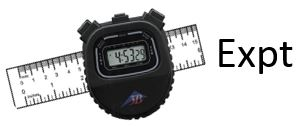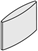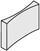Physics Practicals

# PHY385 Module 3: The Thin Lens Equation

PHY385 Module 3 - PHY385 Student Guide Oct. 10, 2016, 4:47 p.m.

##Activity 3.1 – Thin Lens Solution When (so + si) is Fixed - Theortical

An object held a distance so in front of a thin lens with positive focal length f will form a focused image on a viewing screen a distance si beyond the lens if:

$$\begin{eqnarray*} {1 \over s_o} + {1 \over s_i} = {1 \over f} \end{eqnarray*}$$

An object and a viewing screen are held at a fixed distance d, and a focusing lens with positive focal length f is placed part-way between them. In order to form a focused image, the sum of the object and image distances must be equal to d: so + si = d.

1. Combine the thin lens equation with the requirement that so + si = d to eliminate so and solve for si in terms of f and d only.
1. Identify the discriminant of the quadratic equation for Part A.  If the discriminant is negative, then the solution for si will have an imaginary component.  Physically, this means that a focus is impossible and the image will always be blurry. For what condition on d will a focused image be impossible?

Magnification, M, is the ratio of the image size to the object size.  By definition, |M| = hi/ho, where hi is the height of the image measured perpendicular to the optical axis, and ho is the height of the object measured perpendicular to the optical axis. If the image is inverted, M is negative.  For an image formed by a thin lens:

$$\begin{eqnarray*} M = -{s_i \over s_o} \end{eqnarray*}$$

1. Consider a situation where d = 1.0 m, and f = 0.2 m.  What are the two solutions of si ? What is the magnification, M, for the two solutions?

##Activity 3.2 – Thin Lens Solution When (so + si) is Fixed - Experimental

This activity uses optical components clipped to the 2.2m aluminum track.  It is easy to slide these components along the length of the track, and to measure their position using the ruler on the track. Set up the Viewing Screen at 20 cm.  This means the front surface of the white screen should be above the 20 cm mark on the track, and facing down the length of the track where you will be placing other components. Place Lens “A” in the Dynamics Optics Carriage and set up the lens at about 80 cm and the light source with the illuminated crossed arrows pattern at 140 cm, so the pattern is facing toward the lens.  In this case, the distance between the source and the screen, d, is 120 cm.  But you can move the screen or the light source to reduce this.

1. Choose a value of d from the example table below, and set the illuminated pattern and the viewing screen to be at this separation.First slide the lens close to the screen, then slowly slide the lens away from the screen until a clear image of the crossed-arrow object is formed on the screen.Measure the image distance si and the object distance so.Also measure the object size ho and the image size hi.The object size is the distance between two pattern features on the crossed-arrow object, and the image size is the corresponding distance between these features in the image. From measurements of so and si you can predict the magnitude of the magnification, |Mpred| = si / so , and compare with the measured magnification |Mmeas| = hi / ho.Note there may be two very different values of si that give a clear image.[As d decreases there may be only one or even no values of si that give a clear image – just fill in “NO FOCUS” under si in these cases.]

Lens “A”

 d si 1/si so 1/so hi ho |Mmeas| = hi / ho |Mpred| = si / so 120 cm 120 cm 110 cm 110 cm 100 cm 100 cm 90 cm 90 cm 80 cm 80 cm 70 cm 70 cm 60 cm 60 cm 50 cm 50 cm

1. Plot 1/so versus 1/ si and find the best fit line (linear fit).This should give a straight line with the y-intercept equal to 1/f.What is the value of f for this lens?
2. Repeat for Lens B.

Lens “B”

 d si 1/si so 1/so hi ho |Mmeas| = hi / ho |Mpred| = si / so 120 cm 120 cm 110 cm 110 cm 100 cm 100 cm 90 cm 90 cm 80 cm 80 cm 70 cm 70 cm 60 cm 60 cm 50 cm 50 cm
1. Plot 1/so versus 1/ si and find the best fit line (linear fit).  This should give a straight line with the y-intercept equal to 1/f.  What is the value of f for this lens?

##Activity 3.3 – Focal Points in Two Dimensions

This activity uses the “Ray Box” feature of the PASCO Basic Optics Light Source.  Place the light source flat on the table or on your notebook so it is sitting on its four little legs and plug it in.  There is a wheel to select one, three or five parallel rays projected onto the table.  If you place it on your open notebook the rays will be easier to see and you can trace them with a pen or pencil.

You also should have a flat glass convex lens, a flat glass concave lens, and a ruler.

 Converging Lens:Diverging Lens:1. Select the 5-rays and shine them on an open page of your notebook.  Take the converging lens and focus the rays, so that the focal point is on your page.  Sketch the five rays and the exterior shape and position of the lens.  Label the focal point.  Measure the focal length of the lens, which is the distance between the centre of the lens and the focal point for initially parallel rays.
1. Select the 5-rays and shine them on an open page of your notebook.  Take the diverging lens and de-focus the rays.  Leave enough room on the page so that you will be able to sketch the rays backwards to the virtual focal point from which they appear to be emerging.   Sketch the five rays and the exterior shape and position of the lens.  Remove the lens and use a ruler to trace the rays backward to the spot from where they all seem to be emerging.  Label the virtual focal point.  Measure the focal length of the lens, which is related to the distance between the centre of the lens and the virtual focal point for initially parallel rays.
1. Switch the wheel to the red, green and blue thick beams. Using the lenses and these coloured beams, can you create white light?

##Activity 3.4 – Thin lenses: position of objects and images

1- A point source S of light is on the central axis of a thin positive lens. It is at a distance $$l_1$$ in front of the len, and a real image of S appears at P, at  a distance $$l_2$$ from the lens.

A. Is it possible possible to move the lens along the axis to a new location and not finally change the position of S and ? if so, where must the lens be relocated?

B. Draw a diagram.

2- An object on the central axis is 40cm in front of a thin positive lens. Its image appears on a screen 80cm beyond the lens. Now move the lens to a new location on the axis such that the image is again on the screen.

Decribe what happens, if anything, to the size and orientation of the image as a result of the displacement of the lens.

3 - With the previous two problems in mind, imagine a self limiuous object on the central axis of a thin positive lens. The object is a distance d from the screen on which the image appears. Now suppose the lens is moved toward the object to a new location, whereupon the image on the screen is N times larger than it was originally.

Show that the lens has a focal length given by   $$f = \frac{\sqrt{N}d}{(1+\sqrt{N})^2}$$

This activity is extracted fromn Optics by Eugene Hecht, ed 5.   Added by Damya Souami